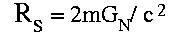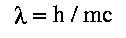QUESTION #191

# Why isn't the electron considered a black hole? It does have mass and its radius is infinitely small, isn't it?

In actuality, we don't know how big the electron is. All of our measurements point to the electron having no size, but we haven't measured down far enough. The electron, if it were a black hole, would have to be smaller than 1x10-57 meters, quite a bit smaller than we've ever measured!

But, another reason that the electron is not considered a black hole, even assuming that its radius is infinitely small, is that it obeys the laws of quantum field theory. Normally, when one speaks about black holes, one is talking about them in terms of Einstein's theory of general relativity. No one is sure how nature merges Einstein's theory with quantum field theory. So we aren't really sure if the idea of a black hole makes sense on distance scales as small as the (possible) radius of the electron.. Our best idea to unify general relativity with quantum field theory is an idea called string theory, but string theory still appears to be a long way from being put to any experimental tests.

The electron (and all truly 'fundamental' particles) are considered to be true mathematical points in the sense that they have no classical spatial extent. This is known, for example in the case of the electron, by performing scattering experiments: the way particles scatter off one another is quite different if the target is represented as a point as opposed to having some finite size. All of the electron scattering experiments done so far are consistent with the hypothesis that the electron is truly a 'point particle.'

According to general relativity all massive objects possess an event horizon known as the Schwarzschild radius. This is a surface in three-dimensional space surrounding the object. Any light rays emitted from within this radius are unable to escape. If an object exists entirely within its Schwarzschild radius then it is referred to as a black hole. This radius grows with the mass of the object according to the formula:Notice that for our sun we obtain a radius of 2.95 kilometers. This is well within the interior of the sun so it is not a black hole. For an electron we would obtain 1.35 x10-51 m. If the electron were a point particle, it seems it would be within even this fantastically small radius and would indeed be a black hole!

However, as a subatomic particle the electron is also a quantum-mechanical object. Recall the wave-particle duality hypothesis of de Broglie. All objects have a wave function which represents the probability of locating that object at a particular point in space. During a collision this wave function momentarily collapses and the particle is truly at one 'point' in space, but it immediately starts to spread out again after the instant of collision. The typical spread of the wave-function of a point particle is given by the Compton wavelength:and this can be considered the true quantum-mechanical 'size' of the object. Notice that this size gets smaller as the mass gets larger. For you or I or the sun this quantum-mechanical size is essentially zero (there's not much uncertainty as to where the sun is!) but for an electron the size is 2.42 x10-12 m. Though still small, this is much, much larger than the Schwarzschild radius. So quantum-mechanically most of the electron is 'outside' its event horizon. That's why it and other subatomic particles are not black holes.
Answered by: Brent Nelson, M.A., Physics Ph.D. student, UC Berkeley